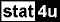#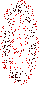LEAST SQUARES METHOD  CURVE FITTING PROGRAM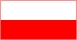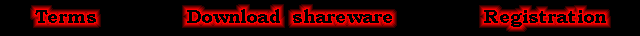Brief description of the program.
LSM program performs the Least Squares Nonlinear Regression Analysis. The method is also called: Curve Fitting, Least Squares Fitting, Least Squares Method, Least Squares Estimation, Least Squares Approximation.
The program fits parameters of curve(s) given analytically to given points.
The name Maximum Likelihood Estimation locates the method used by the program in the appropriate section of statistics.   LSM program can carry out the nonlinear regression analysis:
curve fitting
• in many dimensions,
• with input uncertainties in all axes,
• with input correlation coefficients or covariance matrix, if needed,
• using many curves approximated simultaneously,
curves may be stuck with some of their parameters as well as with some points.
• Tens of basic functions are available (to create any curve equation).
• Goodness of fit can be controlled by the Chi-Square quantity
and the Side-Correlation coefficient.
• Adjusted (estimated) points and their uncertainties can also be shown.

 LSM 2.4  -  the latest version improved in its core algorithm. Now, the LSM program works fast and very precisely.
Nonlinear Least Squares Regression Analysis. Curve Fitting. Nonlinear Weighted Least Squares Estimation Approximation.
Hints
Every parameter must be denoted by a1, a2, a3, ...,
and every point coordinate - by x1, x2, x3, ...
(instead of x ,y, z, ...).

Maximum Likelihood Method. Nonlinear Weighted Least Squares Approximation.
Each function must have the form of an expression obtained by transferring all terms to one side of an equation.
z = a1*x2 + a2*y + a3    with    x3= a1*x1^2 + a2*x2 + a3
and write it in the implicit form  F(x1,x2,x3)=0,  without the "=0" part:     a1*x1^2 + a2*x2 + a3 - x3
Curve Fitting. Least Squares Method. Least Squares Fitting. Least Squares Estimation.
This form enables applying complicated curves that are not a graph of any function, applying more complicated curve equations from which none of variables can be derived explicitly  as well as transforming equations to be always computable. The last case may be useful when some point coordinate or a parameter value being changed during computation  temporarily goes beyond domain boundary.

Nonlinear Least Squares Regression Analysis. Nonlinear Weighted Least Squares Estimation Approximation.
Use the LSM program especially when the point uncertainties are in many axes and they are all known, or point correlation coefficients or covariance matrix are known, or the problem is in more than two dimensions, or the curve is not a graph of any function (e.g. a circle), or the curve is given by an equation from which no variable can be derived, or you use a combination of curves stuck with points or parameters.
Least Squares Method. Nonlinear Weighted Least Squares Approximation.
To control goodness of fit, the Chi-sqr parameter and its standard deviation is calculated on the basis of input uncertainties. Chi-sqr expected value equals the number of freedom degrees (i.e. the number of points minus the number of parameters). If the Chi-sqr value obtained is close to the number of degrees of freedom, it is likely that the model assumed fits the points with point uncertainties. Too large Chi-sqr value may result from inappropriate choice of analytical function form or from assuming point uncertainties to be too small. Too low value may testify to granting the number of curve parameters to be too large or to assuming point uncertainties to be too large.
Weighted Least Squares Fitting. Correlation Coefficient.
To control goodness of fit, the Side-Correlation Coefficient (rsc) is calculated together with its standard deviation. When the measuring points are located randomly on both sides of the fitted curve (surface, hyper-surface), rsc takes on a value that is close to zero. When the points are grouped in big clusters each located on one side of the curve, rsc considerably differs from zero, i.e. is greater then its double standard deviation, or even approaches the unit, thus indicating that the curve is selected incorrectly (maybe more parameters are needed) or the points are correlated (maybe the points in one cluster come from a biased meter). When rsc is negative and considerably differs from zero or even approaches minus one, it is statistically hardly probable (maybe the points have been created with "human hands").
Goodness of fit. Nonlinear Weighted Least Squares Approximation.
If you are sure of your fitting curve and the reciprocals of uncertainties for all points and dimensions, instead of the uncertainties themselves, you can multiply all input (point) and output (parameter) uncertainties by the square root of the ratio of the output Chi-sqr parameter (the GInf menu item) to the number of freedom degrees. Thus you can obtain the best estimates of uncertainties in this case of incomplete information on input uncertainties.
Curve Fitting. Least Squares Fitting. Least Squares Approximation.
The method used in LSM program gives exactly the same values of parameter uncertainties as "other programs" that do not accept X uncertainties,   if in LSM program all input X (or X1, X2, ... for many dimensions) uncertainties are fixed at zero  and input Y uncertainties are such that the output Chi-sqr parameter (the GInf menu item) is equal to the number of degrees of freedom.
Maximum Likelihood Method. Nonlinear Weighted Least Squares Fitting.
Calculation time is significantly longer in case of input covariance matrix that combines also errors of different points (instead of correlation coefficients that combine only errors of coordinates of every point separately). The calculation time is longer also due to the method nonlinearity caused by the assumption of errors in all coordinates of points. Such a regression is nonlinear even in case of fitting a straight line.

More precise description can also be found in readme file in the program package.
LSM program requires MS-DOS or Microsoft Windows (3/95/98/2000/XP).
In case of a straight line and input uncertainties in x and y axes, the LSM-2 program gives exactly the same results, though it does it in a different way, as the last-minute method for a straight line described in:
D. York, N.M. Evensen, M.L. Martinez, J. Delgado, Unified equations for the slope, intercept and standard errors of the best straight line, Am. J. Phys., Vol. 72, No 3.

Exemplary approximation results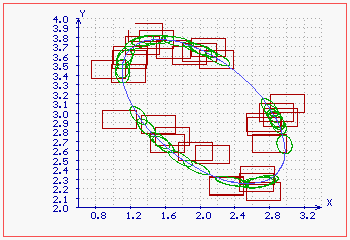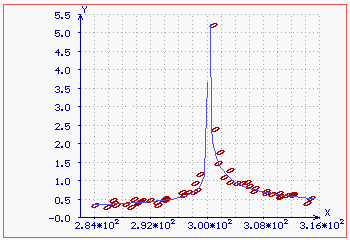Approximating curve - ellipse; Input error rectangles -(red); Output (estimated) error ellipses -(green). Two different approximating curves with common parameter (i.e. asymptote abscissa); Input correlation coefficients - error ellipses (red).

Least Squares Nonlinear Regression Analysis, Curve Fitting, Least Squares Fitting
Each function must have the form of an expression obtained by transferring all terms to one side of equation!
Every parameter must be denoted by a1, a2, a3, ...,
and every point coordinate - by x1, x2, x3, ... (instead of x ,y, z, ...).
Curve Fitting, Least Squares Method, Least Squares Fitting, Nonlinear Weighted Least Squares Estimation Approximation Nonlinear Least Squares Regression Analysis, Nonlinear Weighted Least Squares Approximation Estimation

Least Squares Method, Least Squares Estimation, Least Squares Approximation
 LSM 2.4  -  the last version improved in its core algorithm. Now, the LSM program works fast and very precisely.

 LSM 2.4 Package (0.5 MB)  v. August 2013 go to the Program description go to the Comparison get the Theory

Curve Fitting, Least Squares Fitting, Least Squares Approximation
Installation and use
Unpack the contents of the package to anywhere on the hard disc.
Use  LSM23-demo.exe  as a demo.
Use  LSM24-s.exe  for single precision of calculation and the maximum number of points.
Use  LSM24-r.exe  for real precision of calculation.
Use  LSM24-d.exe  for double precision of calculation and the minimum number of points.
The program doesn't even touch Windows registers.
The program requires 32-bit Windows.
In 64-bit Windows you can install a 16-bit emulator.

Least Squares Estimation, Least Squares Approximation
Least Squares Nonlinear Regression Analysis, Curve Fitting, Maximum Likelihood Estimation
Registering
Since the program is free for non-commercial use now, there is no need to register it.
Nonlinear Least Squares Regression Analysis, Maximum Likelihood Estimation
History
LSM 1   (January 1998) - basic algorithm.
LSM 1.1 (July 2009) - a little modification that allows further iteration after 0.1-standard-uncertainty accuracy has been reached. Also numerical derivatives are calculated more exactly now to be consistent with the modification.
LSM 2   (September 2009) - basic algorithm improvement. Now the program can calculate parameters and adjusted points as well as their uncertainties with an accuracy of the last digit (which is probably needed only to compare with other programs).
LSM 2.1 (December 2009) - the time of calculation has been significantly shortened and the maximum number of points has been increased in the most common case of two-dimensional input data and when different points are not correlated and when different curves (if there are such) are not stuck with some points. The improvement has been achieved by analytical transformation of matrix formulae for output parameters. Also a possibility of entering correlation coefficients for coordinates of each point has been added in case of two-dimensional data so the covariance matrix is necessary only when different points are correlated. The mouse wins back its functionality. Some little improvements has been made.
LSM 2.2 (January 2010) - the time of calculation is short and the maximum number of points is increased in all dimension cases, not only in 2D case. Some little improvements has also been made.
LSM 2.3 (May 2010) - Side-Correlation Coefficient has been added to control additionally goodness of fit. Also, possibility of calculating best estimates of uncertainties in case of incomplete information on input uncertainties has been added. Some little improvements has also been made (like saving the graph to a bitmap file - Ctrl S) and a few bugs in input-output procedures has been removed.
LSM 2.4 (December 2010) - the plot does not hang up when the plotted range exceeds the function domain. Max. number of points has been increased from 3275 (in 2D case) to
8190 - LSM24-s  version,
5460 - LSM24-r  version,
4095 - LSM24-d  version.
Max. number of points proportionally decreases for greater dimension number.
A bug in Which menu has been removed (2011.08.20).
Curve Fitting, Least Squares Method, Least Squares Fitting

 Please forward questions or suggestions to the author: janand@prz.edu.pl affiliation (in Polish) J. A. Mamczur, PhD Institute of Physics Rzeszow University of Technology Al. Powstancow Warszawy 6 35-959 Rzeszow, POLAND

Least Squares Method, Least Squares Estimation Curve Fitting, Least Squares Fitting, Least Squares Approximation
Back to the top
Since the program is free for non-commercial use now, the restrictions concerning non-commercial using and spreading the program are canceled.

BY DOWNLOADING LSM PROGRAM YOU ARE AGREEING TO BIND YOUR COMPANY AND YOURSELF TO THE TERMS OF THIS AGREEMENT. A LICENSE TO USE THE SOFTWARE WILL NOT BE GRANTED UNLESS YOU AND YOUR COMPANY AGREE TO THE TERMS OF THIS AGREEMENT. DOWNLOADING AND INSTALLING THE PROGRAM WILL BE AN IRREVOCABLE ACCEPTANCE OF THE TERMS OF THIS AGREEMENT.

YOU MAY:
1. use the registered version of the Program for evaluation purposes on a single computer or network, and only by a single user at a time regardless of the number of original copies of the Program included with the Program. If you wish to use the Program for more users, you will need an additional license for each user;
2. make one copy of the registered version of the Program for archive or back-up purposes.

YOU MAY NOT:
1. use the Program or make copies of it except as permitted in this License;
2. translate, reverse engineer, decompile or disassemble the Program, except to the extent the foregoing restriction is expressly prohibited by applicable law;
3. rent, lease, assign or transfer the Program;
4. modify the Program or merge all or any part of the Program in another program.
5. make both the registered version of the Program and the registration code available over a network for multiple users, access, distribute them in any form or provide them in conjunction with any other product.

Disclaimers
LSM Program is provided without warranty of any kind, express or implied, and the user assumes the entire risk of using it.
The author does not assume responsibility for any expense, damage or loss caused by your use of this software, however it comes down.
All the registered trademarks used herein are registered.
This notification is given in lieu of any specific list of trademarks and their owners, which would not be as inclusive and would probably take a lot longer to type.

If you register software, we will assume that you are doing so having tested the shareware version and ascertained that it's suitable for your hardware and requirements.
We cannot provide refunds for shareware registration if you subsequently change your mind.

The shareware version is limited regarding the maximum number of used points.
Nothing more in the shareware version is suppressed or crippled nor will suddenly stop working after a predetermined period of time. If something isn't working as you think it should in the shareware, this will not change in the registered version.

No portion of the documentation for this software or any of its attendant files may be reproduced in whole or in part in any medium or form of transmission.

Registering
Registering this software buys you a single-user license to use it in perpetuity, subject to the terms discussed herein. This means that your registered software can only be used by one person at a time. You might, for example, have a copy on your computer at home and a copy on your computer at work. If you have taken reasonable precautions to ensure that no one else will use your software on one machine while you're working with the other, your use of the registered software is in keeping with the license you have purchased.
A single-user license does not permit you to make the registered software available over a network for multiple users to access, to distribute it in any form or to provide it in conjunction with any other product.
This license extends only to the registered software itself, and in no way affects any files or documents you might create with it.
The registered cost of this software does not include payment for technical support.

Since the program is free now, the restrictions concerning non-commercial spreading and using of the program are canceled.